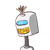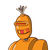# If 10 men can build a wall in 5 days then in how many days 5 men can build the same wall

If 10 men can build a wall in 5 days then in how many days 5 men can build the same wall

### 2 thoughts on “If 10 men can build a wall in 5 days then in how many days 5 men can build the same wall”

1.[tex]\huge\red{Q}\green{ue}\blue{St}\orange{i}\pink{o}\red{n}[/tex]

If 10 men can build a wall in 5 days then in how many days 5 men can build the same wall

[tex]\huge\tt\pink{Ɑղʂⱳҽɾ…♡}[/tex]

=x =10x50=50

=50 divided by 5 which gives 10 as a final result

Answer-5 men can build the same wall in 10 days...

[tex]\huge \mathfrak \red{❥Thank You!}[/tex]

2.